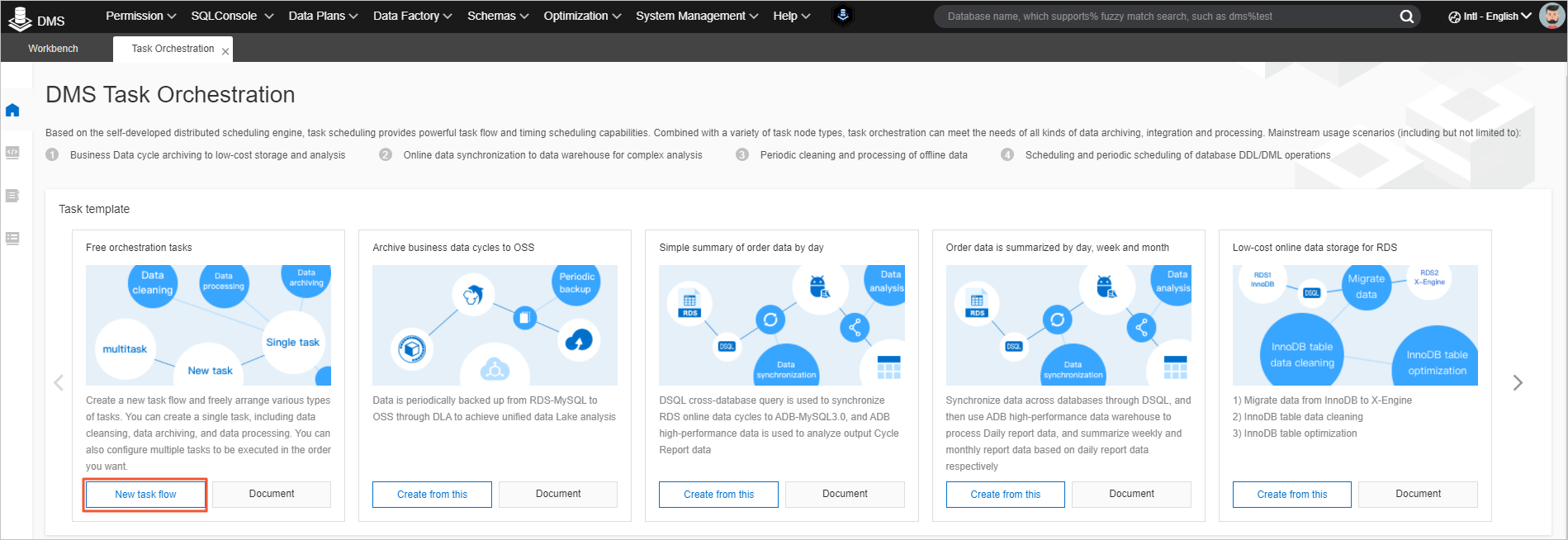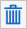The task orchestration feature of Data Management (DMS) supports multiple time variables. You can reference predefined time variables in a task or a task flow. This feature simplifies the SQL statements and improves development efficiency.

## Procedure

1. Log on to the DMS console.3. In the New Task Flow dialog box, set the Task Flow Name and Description parameters and click OK.
4. On the task orchestration tab of the task flow that you created, click the blank area on the canvas.
5. In the right-side pane, click the Variables tab.
Note You can also configure variables for a task. The variables that are configured for a task flow and those for a task have the following differences:
• If you configure variables for a task flow, you can reference the variables in each task of the task flow.
• If you configure variables for a task, you can reference the variables only in the task.
6. Set the Variable Name and Variable Rule parameters as required.
Parameter Description
Variable Name The name of the custom variable.
`bizdate` is the only default system variable, which indicates the previous day of the day when a task is run. The value of the bizdate variable is in the `yyyy-MM-dd` format. For example, if the task is run on August 15, 2019, the value of the `bizdate` variable is 2019-08-14.
Note To delete a configured variable, click theicon.
Variable Rule The time format of the custom variable. All custom variables are defined based on the value of the `bizdate` variable. For more information, see Time formats.
You can also configure an offset to the value of the bizdate variable. The plus sign ( `+`) indicates the backward offset time and the minus sign ( `-`) indicates the forward offset time. For example, `- 6 Month` indicates the point in time six months before a task is run.
Note After you configure the variable, you can reference the variable in the `\${Variable name}` format in SQL statements. On the Content tab of a task node, you can click SQLPreview to check whether the variable is configured as expected.
7. Click Save.

## Time formats

The following table describes the time variables that can be used to define the time format of a custom variable.

Time variable Description Sample format Sample value
Year
• y: the year of the day when the task is run. y or yyyy indicates the year in the four-digit format. yy indicates the last two digits of the year.
• Y: the year of the last day in the week when the task is run. The last day of the week is Sunday.
yyyy 2021
Month M: the month of the year when the task is run. Valid values of M: [1,12]. Valid values of MM: [01,12]. MM 08
Week
• w: the week of the year when the task is run. Valid values of w: [1,52]. Valid values of ww: [01,52].
• W: the week of the month when the task is run. Valid values: [1,5].
ww 13
Day
• D: the day of the year when the task is run. Valid values of D: [1,365]. Valid values of DD: [01,365]. Valid values of DDD: [001,365].
• d: the day of the month when the task is run. Valid values of d: [1,31]. Valid values of dd: [01,31].
D 360
Day of the week
• E: the day of the week when the task is run. Valid values: Monday to Sunday.
• e: the number of the day in the week when the task is run. Valid values: [1,7]. A value of 1 indicates Monday.
Note To count Sunday as the first day of the week, you can set the offset to "+ 1 Day".
e 1
Ante meridiem (AM) or post meridiem (PM) a: indicates whether the point in time when the task is run is before or after the midday. If the time in point is from 00:00 to 11:59, the return value is AM. If the time in point is from 12:00 to 23:59, the return value is PM. a AM
Hour
• H: the hour of the day when the task is run. A value of 0 indicates the first hour of the day. Valid values of H: [0,23]. Valid values of HH: [00,23].
• h: the hour of the half day when the task is run. A value of 1 indicates the first hour of the half day. Valid values of h: [1,12]. Valid values of hh: [01,12].
• K: the hour of the half day when the task is run. A value of 0 indicates the first hour of the half day. Valid values of K: [0,11]. Valid values of KK: [00,11].
• k: the hour of the day when the task is run. A value of 1 indicates the first hour of the day. Valid values of k: [1,24]. Valid values of kk: [01,24].
HH 10
Minute m: the minute of the hour when the task is run. Valid values of m: [0,59]. Valid values of mm: [00,59] m 27
Second
• s: the second of the minute when the task is run.
• S: the millisecond of the minute when the task is run.
ss 08
Time zone z: the time zone. z UTC+08:00
The following table describes the sample time formats that use multiple time variables.
Sample format Sample value
`yyyy-MM-dd` 2019-08-02
`HH:mm:ss` 11:05:21
`yyyyMMdd HH:mm:ss` 20190802 11:05:21
`yyyyMM01` 20190801